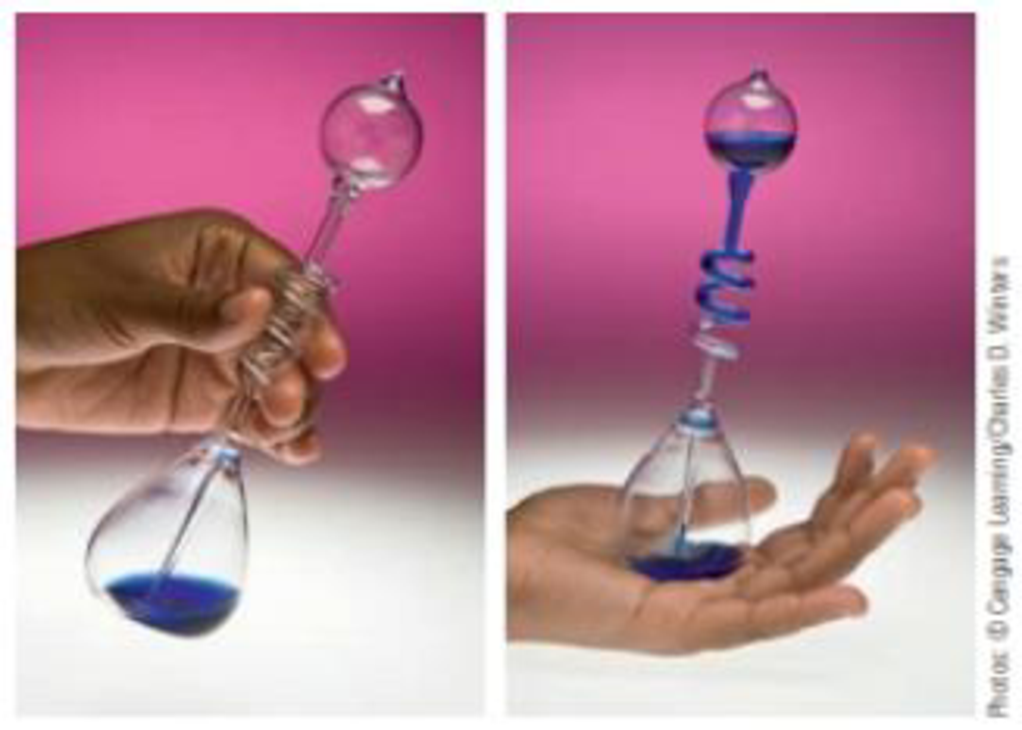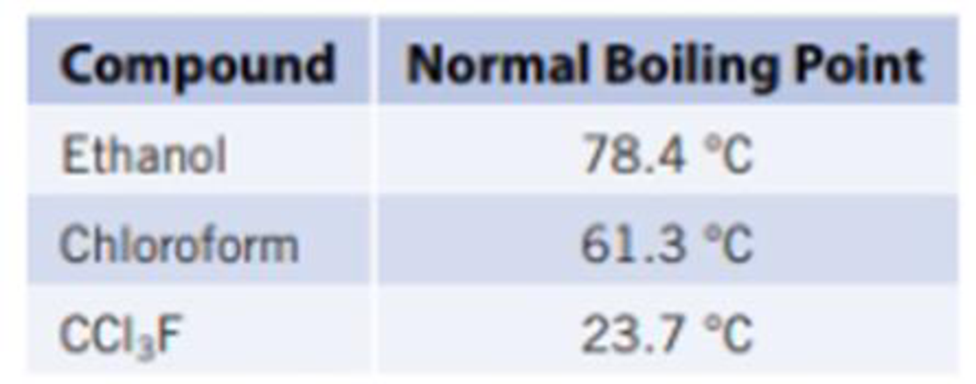Chapter 11, Problem 40IL

Chapter
Section
Textbook Problem

A “hand boiler” can be purchased in toy stores or at science supply companies. If you cup your hand around the bottom bulb, the volatile liquid in the boiler boils, and the liquid moves to the upper chamber. (a) Using your knowledge of kinetic molecular theory and intermolecular forces, explain how the hand boiler works.(b) Which of the following liquids would be best to use in the hand boiler? Explain.(a)

Interpretation Introduction

Interpretation: The working of hand boiler has to be determined using kinetic molecular theory and intermolecular forces.

Concept Introduction:

Kinetic-molecular theory of gases:

Properties of gases are different from solids and liquids. When pressure is applied to gases, they are easily compressed to smaller volume and they have low densities. These properties can be explained by a theory called kinetic-molecular theory.

The four assumptions of this theory are given below:

• Gases are made up of particles like atoms or molecules which randomly moves and there will be no attractive forces between them.
• Comparing the space occupied by the gas particles and the space between particles, the former is much smaller than the latter.
• The average kinetic energy of gas particles and the Kelvin temperature is proportional.
• There is an elastic collision between gas particles or with the wall of their container and thus no energy is lost during collision and total kinetic energy of the particles is constant.

According to this theory, the random motion of the gases allows it to mix together quickly and the gas particles are made up of atoms or molecules with no force of attraction between them

Intermolecular force: The attractive force that withholds two molecules is called as intermolecular force. The influence of intermolecular forces depends on molar mass and the functional group present in the molecule.

Explanation

The liquid is kept in a closed container. The liquid absorbs temperature when it is kept in hand. This temperature is enough to converts molecules to gaseous state since the liquid used has low intermolecular forces...

(b)

Interpretation Introduction

Interpretation: The best liquid to use in the hand boiler has to be identified.

Concept Introduction:

Intermolecular force: The attractive force that withholds two molecules is called as intermolecular force. The influence of intermolecular forces depends on molar mass and the functional group present in the molecule.

If intermolecular force is small the vapor pressure of the substance is high and the boiling point will be low.

Still sussing out bartleby?

Check out a sample textbook solution.

See a sample solution

The Solution to Your Study Problems

Bartleby provides explanations to thousands of textbook problems written by our experts, many with advanced degrees!

Get Started

Find more solutions based on key concepts